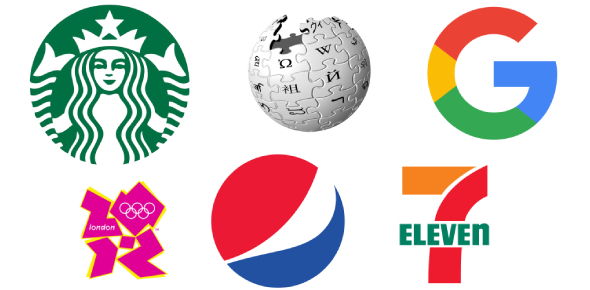# Technical MCQ By Mcs'17

60 Questions | Total Attempts: 91SettingsCreate your own QuizConducted by Math Computing Society

• 1.
Which of the following relational algebra operations do not require the participating tables to be union-compatible?
• A.

Union

• B.

Intersection

• C.

Difference

• D.

Join

• 2.
Which of the following is not a property of transactions?
• A.

Atomicity

• B.

Concurrency

• C.

Isolation

• D.

Durability

• 3.
Relational Algebra does not have
• A.

Selection operator

• B.

Projection operator

• C.

Aggregation operators

• D.

Division operator

• 4.
Checkpoints are a part of
• A.

Recovery measures

• B.

Security measures.

• C.

Concurrency measures

• D.

Authorization measures.

• 5.
Tree structures are used to store data in
• A.

Network model

• B.

Relational model

• C.

Hierarchical model

• D.

File based system

• 6.
The language that requires a user to specify the data to be retrieved without specifying exactly how to get it is
• A.

Procedural DML

• B.

Non-Procedural DML

• C.

Procedural DDL

• D.

Non-Procedural DDL

• 7.
Precedence graphs help to find a AC14/AT11 Database Management Systems 2
• A.

Serializable schedule

• B.

Recoverable schedule

• C.

• D.

• 8.
The rule that a value of a foreign key must appear as a value of some specific table is called
• A.

Referential constraint

• B.

Index.

• C.

Integrity constraint

• D.

Functional dependency.

• 9.
The clause in SQL that specifies that the query result should be sorted in ascending or descending order based on the values of one or more columns is
• A.

View

• B.

Order by

• C.

Group by

• D.

Having

• 10.
What is a disjoint less constraint?
• A.

It requires that an entity belongs to no more than one level entity set.

• B.

The same entity may belong to more than one level.

• C.

The database must contain an unmatched foreign key value.

• D.

An entity can be joined with another entity in the same level entity set.

• 11.
According to the levels of abstraction, the schema at the intermediate level is called
• A.

Logical schema

• B.

Physical schema

• C.

Subschema

• D.

None of the above

• 12.
It is an abstraction through which relationships are treated as higher level entities
• A.

Generalization

• B.

Specialization

• C.

Aggregation

• D.

Inheritance

• 13.
A relation is in ____________ if an attribute of a composite key is dependent on an attribute of other composite key.
• A.

2NF

• B.

3NF

• C.

BCNF

• D.

1NF

• 14.
What is data integrity?
• A.

It is the data contained in database that is non redundant.

• B.

It is the data contained in database that is accurate and consistent.

• C.

It is the data contained in database that is secured.

• D.

It is the data contained in database that is shared.

• 15.
What are the desirable properties of a decomposition
• A.

Partition constraint

• B.

Dependency preservation

• C.

Redundancy

• D.

Security.

• 16.
In an E-R diagram double lines indicate
• A.

Total participation

• B.

Multiple participation.

• C.

Cardinality N

• D.

None of the above.

• 17.
The operation which is not considered a basic operation of relational algebra is
• A.

Join

• B.

Selection

• C.

Union

• D.

Cross product

• 18.
Fifth Normal form is concerned with
• A.

Functional dependency

• B.

Multivalued dependency

• C.

Join dependency

• D.

Domain-key.

• 19.
Block-interleaved distributed parity is RAID level
• A.

2

• B.

3

• C.

4

• D.

5

• 20.
Immediate database modification technique uses
• A.

Both undo and redo

• B.

Undo but no redo

• C.

Redo but no undo

• D.

Neither undo nor redo.

• 21.
What will be output if you will compile and execute the following c code? #define message "union is\ power of c" void main() { clrscr(); printf("%s",message); getch(); }
• A.

Union is power of c

• B.

Union is power of c

• C.

union is Power of c

• D.

Compiler error

• E.

None of these

• 22.
What will be output if you will compile and execute the following c code? #define call(x) #x void main(){ printf("%s",call(c/c++)); }
• A.

C

• B.

C++

• C.

#c/c++

• D.

C/c++

• E.

Compiler error

• 23.
What will be output if you will compile and execute the following c code? converted by Web2PDFConvert.com void main() { if(printf("cquestionbank")) printf("I know c"); else printf("I know c++"); }
• A.

I know c

• B.

I know c++

• C.

cquestionbankI know c

• D.

cquestionbankI know c++

• E.

Compiler error

• 24.
Int y = {6, 7, 8, 9}; int *ptr = y + 2; printf("%d\n", ptr[ 1 ] ); What isprinted when the sample code above is executed? a) 6 b) 7 c) 8 d) 9 Ans: d 27. int i = 4; switch (i) { default: ; case 3: i += 5; if ( i == 8) { i++; if (i == 9) break; i *= 2; } i -= 4; break; case 8: i += 5; break; } printf("i = %d\n", i); What will the output of the sample code above be?
• A.

i = 5

• B.

i = 8

• C.

i = 9

• D.

i = 10

• 25.
What will be the value of `a` after the following code is executed #define square(x) x*x a = square(2+3)
• A.

25

• B.

13

• C.

11

• D.

10

Related TopicsBack to top
×

Wait!
Here's an interesting quiz for you.